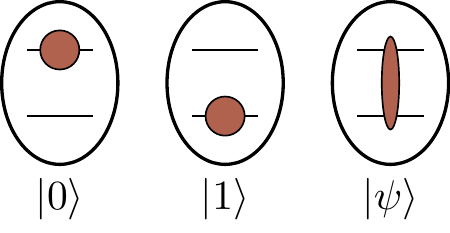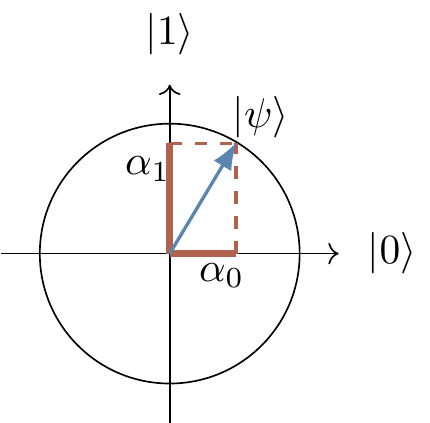## 2.3 Quantum gates and circuits

Atoms, trapped ions, molecules, nuclear spins and many other quantum objects, which we call qubits, can be used to implement simple quantum interference (something which we have still yet to explain), and hence simple quantum computation. There is no need to learn about physics behind these diverse technologies if all you want is to understand the basics of quantum computation. We may now conveniently forget about any specific experimental realisation of a qubit and just remember that any manipulations on qubits have to be performed by physically admissible operations, and that such operations are represented by unitary transformations.

A quantum (logic) gate is a device which performs a fixed unitary operation on selected qubits in a fixed period of time, and a quantum circuit is a device consisting of quantum logic gates whose computational steps are synchronised in time.

The size of such a circuit is the number of gates it contains. The gates in a circuit can be divided into layers, where the gates in the same layer operate at the same time, and the number of such layers is called the depth of a circuit.

Some unitary U acting on a single qubit is represented diagrammatically asThis diagram should be read from left to right. The horizontal line represents a qubit that is inertly carried from one quantum operation to another. We often call this line a quantum wire. The wire may describe translation in space (e.g. atoms travelling through cavities) or translation in time (e.g. a sequence of operations performed on a trapped ion). A sequence of two gates acting on the same qubit, say U followed by V, is represented byand is described by the matrix product VU (note the order in which we multiply the matrices).